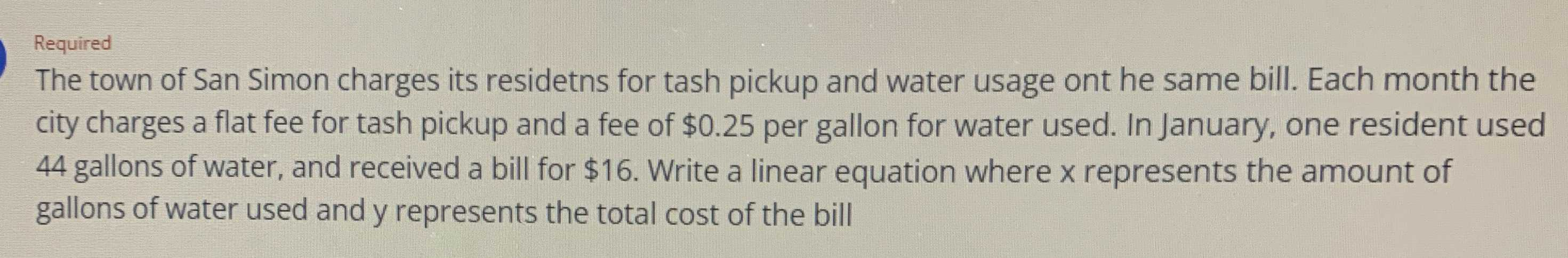### Still have math questions?

Algebra
QuestionThe town of San Simon charges its residetns for tash pickup and water usage ont he same bill. Each month the city charges a flat fee for tash pickup and a fee of $$\ 0.25$$ per gallon for water used. In January, one resident used

$$44$$ gallons of water, and received a bill for $$\ 16$$ . Write a linear equation where $$x$$ represents the amount of gallons of water used and y represents the total cost of the bill

$$y= 0.25x+ 5$$